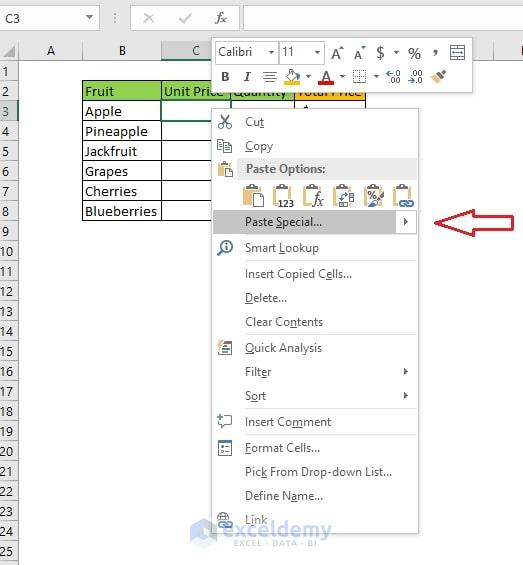# How to Link Data in Excel from One Sheet to Another (4 Ways)MS Excel affords cells in one worksheet connected to cells in other worksheets in the equivalent or separate workbook. In our daily work, we may need these types of things to do. This article will show you how to link data in excel from one sheet to another using various methods.

## 4 Ways to Link Data in Excel

### 1. How to Link Data in Excel from One Sheet to Another, Which are in Different Workbooks.

For showing this process, let’s assume we have three workbooks: fruits unit price, fruits quantity, and fruits total price.For finding the total price for each fruit, we need to link data one with another. I will show how to connect the data Fruits_Total_Price workbook with the other two Fruits_Unit_Price and Fruits_Quantity workbook. We will use the =C3*D3 formula in the E column to find each fruit’s total price.Step 1: Open the Fruits_Unit_Price file and select the Unit price column.Step 2: Right-click on it and select copy or use keyboard shortcut Ctrl+C.Step 3: Open the Fruit_Total_Price file and select the first cell of the Unit Price column.Step 4: Right-click on it and select the Paste Special option.

####Step 5:  Select the Paste link option.Step 6: All the cells will be copied from Fruits_Unit_Price, and the formula will be like this picture.Step 7: Do the same process for the Quantity column to copy from the Fruits_Quantity file. Also, the Total Price will be calculated after that for each fruit.These are the steps for linking data to different files in Excel. Here the formula is generated automatically, but we can input the formulas manually.

Read More: How to Link Multiple Cells from Another Worksheet in Excel (5 Easy Ways)

### 2. How to link data in Excel from one sheet to another, from the same Workbook.

Let’s consider the same example above in different ways. Instead of having different workbooks, we have only one file name Total_Fruits_Price.Inside this workbook, we have three different sheets, separate workbooks in the previous process like Fruits_Total_Price, Fruits_Unit_Price, Fruits_Quantity.Now we will calculate the same total price for each fruit by linking data with other worksheets using the formula =D6*E6 in the F column.

Step 1: Open the Fruits_Unit_Price sheet and select the Unit Price column.Step 2: Right Click on it and select copy or use keyboard shortcut Ctrl+C.Step 3: Go back to Fruits_Total_Price sheets and select the first cell of Unit Price.Step 4: Right-click on it and select Paste Special option.Step 5: Select the Paste Link option.Step 6: Then, you will see all the Unit price is copied from Fruits_Unit_Price is copied and linked to Fruits_Total_Price’s Unit_Price column. And the be a formula like this picture.Step 7: Same Way, we can copy the Quantity from the Fruits_Quantity sheet, and after that, it will be like this. Also, the Total Price will be calculated automatically.Read More: How to Link Cell to Another Sheet in Excel (7 Methods)

### 3. How to Link Data by Entering Formula Manually

For linking data, we enter the formula manually. Suppose we consider the above example. We can connect the data manually. Here are the steps:

Step 1: Select the desired cell which will contain the linked data and type = (Equal) signStep 2: Go to the workbook from where you want to link the data. Like here Fruis_Unit_Price workbook. And select any cell and press enter.Step 3: After pressing enter, the selected value will be copied to the destination.Read More: How to Link Sheets in Excel with a Formula (4 Methods)

### 4. How to Link Data Based on Condition

Sometimes we may need to link data based on conditions for different purposes. In MS-Excel, we can manipulate our linking data depending on various conditions. For example, let’s have two sheets in one workbook, Sheet1 and Sheet2. Sheet1 has some values, and we want to get the data from Sheet1 to Sheet2, which are greater than or equal to 100.

Step 1: Enter the data in Sheet1Step 2:  Go to sheet2 and enter the formula `=IF(Sheet1!C3>=100,Sheet1!C3,"")` in the first cell.Step 3: Copy down the formula, and all values greater than or equal to 100 will be shown.Read More: Transfer Specific Data from One Worksheet to Another for Reports

## Importance of Data Linking in Excel

Generating links can provide help for maintaining the same data in multiple sheets. This saves our valuable time, diminishes errors, and develops data integration for various purposes. As for the above example, if we need to update the total fruit price, we need to input every worksheet or file. We can easily manage data changes using this data linking procedure.

It is always efficient and faster than manual copy over data linking. For the above example, I need to update the Unit Price of any fruit then. If I update the primary Fruit_Unit_Price workbooks, then automatically, all the other workbooks Fruits_Total_Price will also be changed accordingly. Here is the explanation for this. It is workable for both the same or different workbooks in Excel. I am showing the example in other workbooks.

Step 1: Change any fruit’s Unit Price. I have changed the Cherries Unit Price from \$14 to \$9.Step 2: Go to Fruits_Total_Price, and here the price of the Cherries will also be updated, and the Total Price will be calculated correctly.## Conclusion

This is how to copy or link data in MS Excel. Here I have focused on two ways of data linking. Both processes are broadly discussed and show the process with a proper screenshot. Also discussed the importance and the actual benefit of using data linking in Excel.